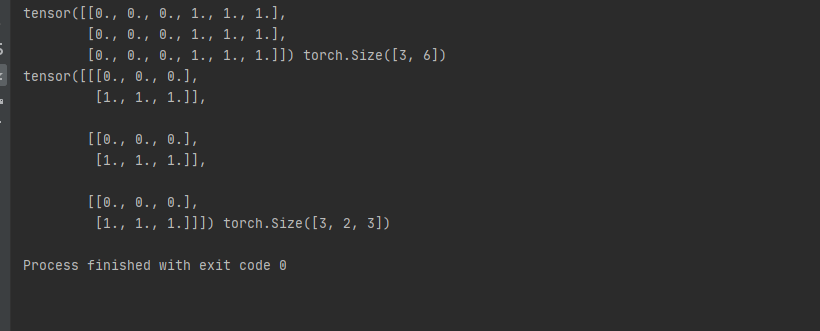## pytorch(3)torch.cat()和torch.stack()

2020/12/5 10:34:06 文章标签:

在进行cv相关实验中我们用的比较多的都是torch.cat()和torch.stack()函数。其中cat()函数的功能是在当前维度进行数据的拼接。stack()函数首先将当前维度及其以后维度的数据向后移动一位，将该位置的大小修改为1然后在进行拼接。这两个函数的区别是cat()函数不会进行维度扩展，stack()函数会进行维度扩展。以下从代码角度去理解这两个函数。

``````import numpy as np
import torch

# 创建两个张量并将其cat到一起。
def create_cat():
t1 = torch.zeros((3, 3))
t2 = torch.ones((3, 3))
t_cat = torch.cat([t1, t2], dim=1)
print(t_cat,t_cat.shape)

# 创建两个张量并将其stack到一起。
def create_stack():
t1 = torch.zeros((3,3))
t2 = torch.ones((3,3))
t_stack = torch.stack([t1,t2],dim=1)
print(t_stack,t_stack.shape)

if __name__ == '__main__':
create_cat()
create_stack()

``````暂无相关的数据...